# Logistic Regression with Minitab

### What is Logistic Regression?

Logistic regression is a statistical method to predict the probability of an event occurring by fitting the data to a logistic curve using logistic function. The regression analysis used for predicting the outcome of a categorical dependent variable, based on one or more predictor variables. The logistic function used to model the probabilities describes the possible outcome of a single trial as a function of explanatory variables. The dependent variable in a logistic regression can be binary (e.g. 1/0, yes/no, pass/fail), nominal (blue/yellow/green), or ordinal (satisfied/neutral/dissatisfied). The independent variables can be either continuous or discrete.

### Logistic Function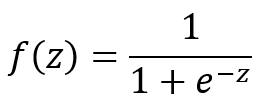Where: z can be any value ranging from negative infinity to positive infinity.
The value of f(z) ranges from 0 to 1, which matches exactly the nature of probability (i.e., 0 ≤ P ≤ 1).
Logistic Regression Equation
Based on the logistic function,we define f(z) as the probability of an event occurring and z is the weighted sum of the significant predictive variables.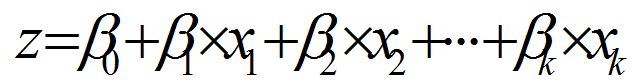Where: Z represents the weighted sum of all of the predictive variables.

### Logistic Regression

Another of way of representing f(z) is by replacing the z with the sum of the predictive variables.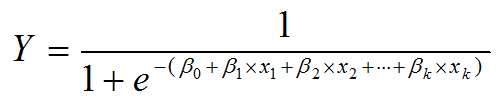Where: Y is the probability of an event occurring and x’s are the significant predictors.
Notes:

• When building the regression model, we use the actual Y, which is discrete (e.g. binary, nominal, ordinal).
• After completing building the model, the fitted Y calculated using the logistic regression equation is the probability ranging from 0 to 1. To transfer the probability back to the discrete value, we need SMEs’ inputs to select the probability cut point.

### Logistic Curve

The logistic curve for binary logistic regression with one continuous predictor is illustrated by the following Figure.

###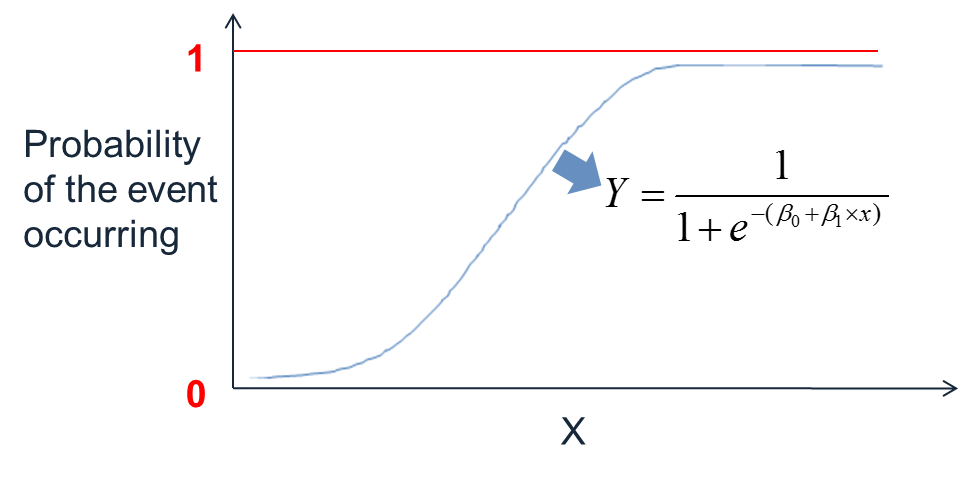Odds

Odds is the probability of an event occurring divided by the probability of the event not occurring.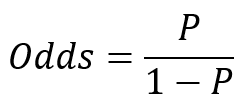Odds range from 0 to positive infinity.
Probability can be calculated using odds.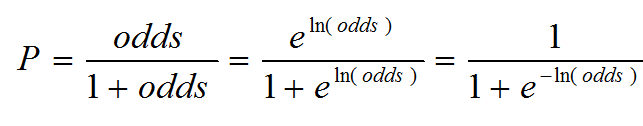Because probability can be expressed by the odds, and we can express probability through the logistic function, we can equate probability, odds, and ultimately the sum of the independent variables.
Since in logistic regression model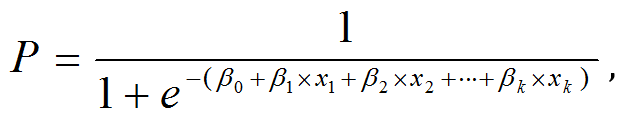therefore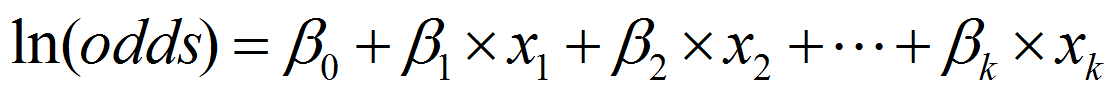### Three Types of Logistic Regression

• Binary Logistic Regression
• Binary response variable
• Example: yes/no, pass/fail, female/male
• Nominal Logistic Regression
• Nominal response variable
• Example: set of colors, set of countries
• Ordinal Logistic Regression
• Ordinal response variable
• Example: satisfied/neutral/dissatisfied

All three logistic regression models can use multiple continuous or discrete independent variables and can be developed in Minitab using the same steps.

### How to Run a Logistic Regression in Minitab

Case Study: We want to build a logistic regression model using the potential factors to predict the probability that the person measured is female or male.
Data File: “Logistic Regression” tab in “Sample Data.xlsx”

Response and potential factors

• Response (Y): Female/Male
• Potential Factors (Xs):
• Age
• Weight
• Oxy
• Runtime
• RunPulse
• RstPulse
• MaxPulse

Step 1:

1. Click Stat → Regression → Binary Logistic Regression→ Fit Binary Logistic Model
2. A new window named “Binary Logistic Regression” appears.
3. Click into the blank box next to “Response” and all the variables pop up in the list box on the left.
4. Select “Sex” as the “Response.”
5. Select “Age”, “Weight”, “Oxy”, “Runtime”, “RunPulse”, “RstPulse”, “MaxPulse” as “Continuous predictors.”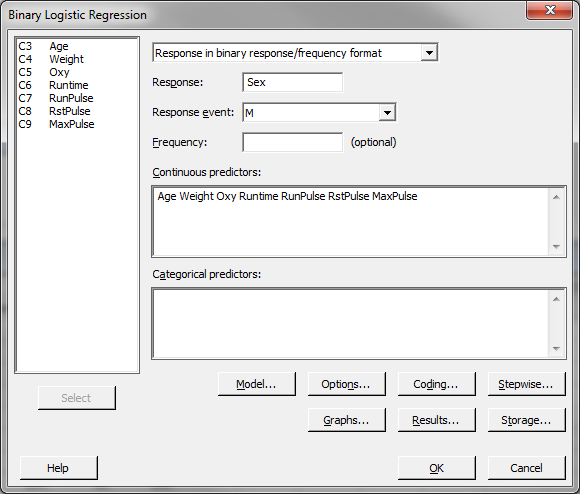6. Click “OK.”
7. The results of the logistic regression model appear in session window.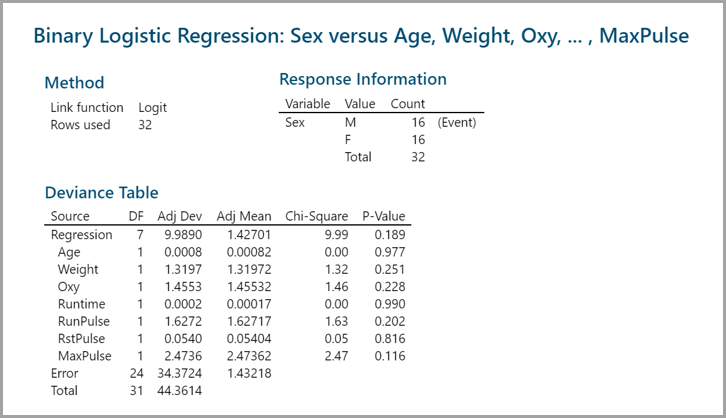Step 2:

1. Check the p-values of all the independent variables in the model.
2. Remove the insignificant independent variables one at a time from the model and rerun the model.
3. Repeat step 2.1 until all the independent variables in the model are statistically significant.

Since the p-values of all the independent variables are higher than the alpha level (0.05), we need to remove the insignificant independent variables one at a time from the model, starting with the highest p-value. Runtime has the highest p-value (0.990), so it will be removed from the model first.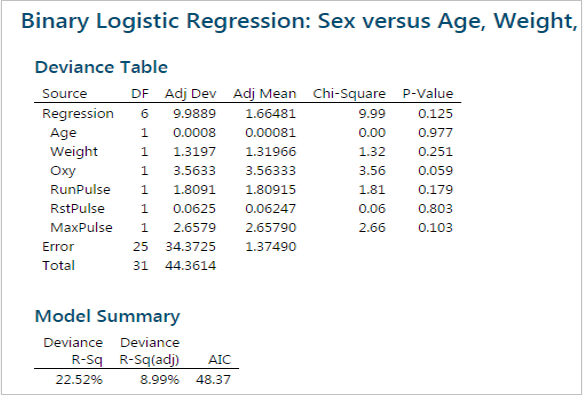After removing Runtime from the model, the p-values of all the independent variables are still higher than the alpha level (0.05). We need to continue removing the insignificant independent variables one at a time, continuing with the highest p-value. Age has the highest p-value (0.977), so it will be removed from the model next.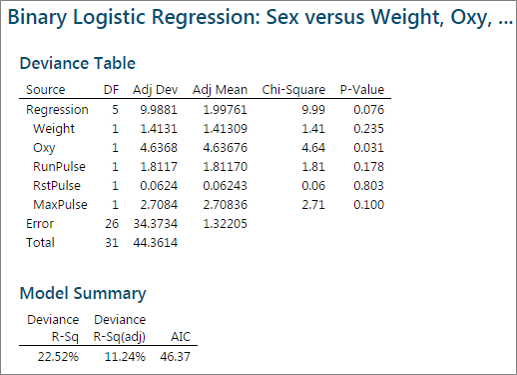After removing both Age and RunTime from the model, the p-values of the remaining independent variables are still higher than the alpha level (0.05). We need to continue successively removing the insignificant independent variables. Continue with the next highest p-value. RstPulse has the highest p-value (0.803) of the remaining variables, it will be removed next.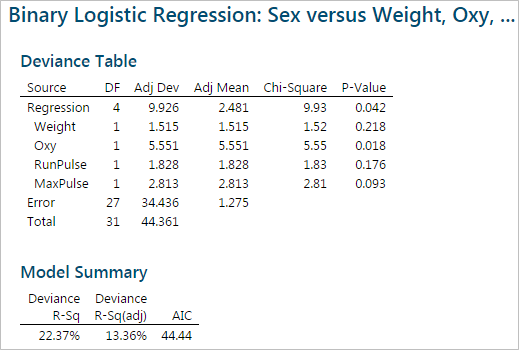After removing RstPulse from the model, the p-values of all the independent variables are still higher than the alpha level (0.05). Continue removing the insignificant independent variables. Weight has the highest p-value (0.218) of the remaining variables, it will be removed next.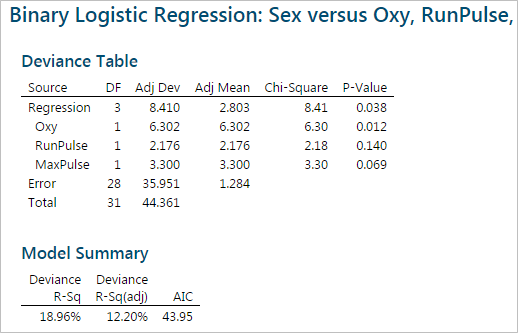After removing Weight from the model, the p-values of the remaining three independent variables are still higher than the alpha level (0.05). Once again, remove the next highest p-value. RunPulse with a p-value of 0.140 should be next.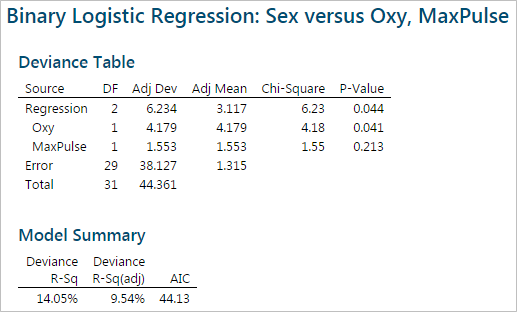After removing RunPulse from the model, the last two p-values are still higher than the alpha level (0.05). We need to remove one more insignificant variable, it will be MaxPulse with a p-value of 0.0755.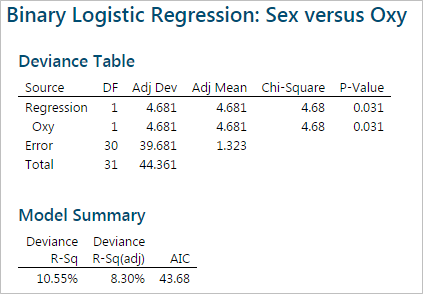After removing MaxPulse from the model, the p-value of the only independent variable “Oxy” is lower than the alpha level (0.05). There is no need to remove “Oxy” from the model.

Step 3:

Analyze the binary logistic report in the session window and check the performance of the logistic regression model. The p-value here is 0.031, smaller than alpha level (0.05). We conclude that at least one of the slope coefficients is not equal to zero. The p-value of the “Goodness-of-Fit” tests are all higher than alpha level (0.05). We conclude that the model fits the data.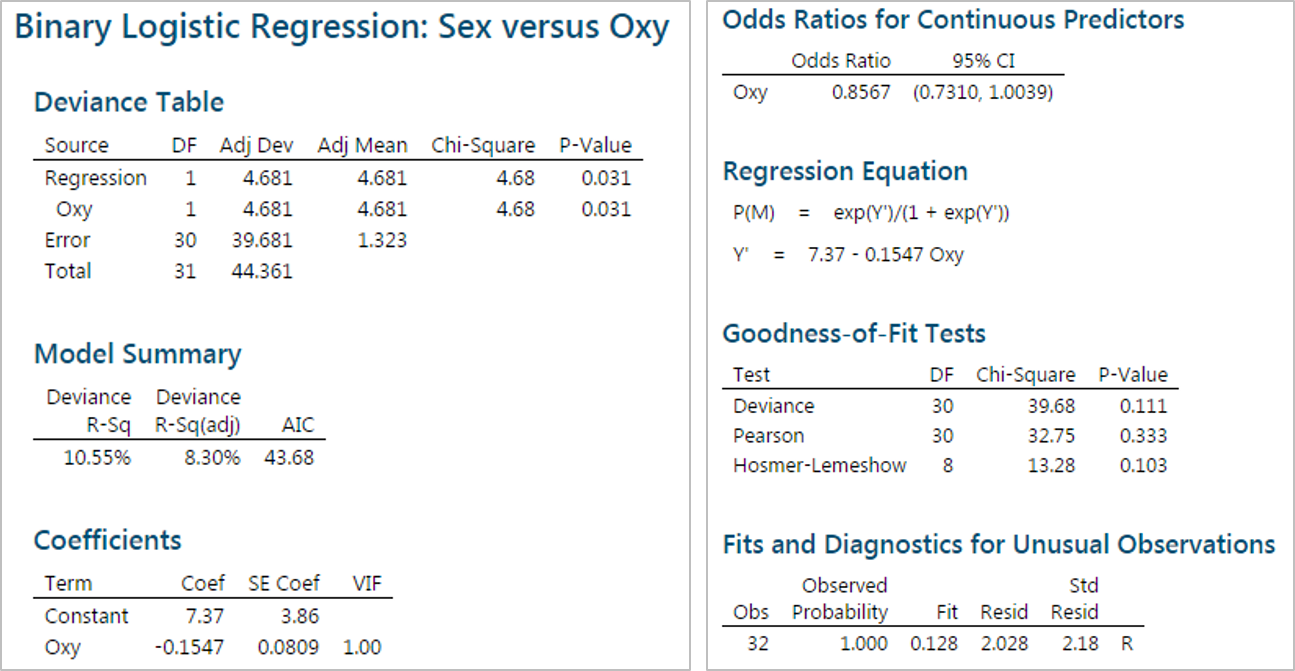Step 4: Get the predicted probabilities of the event (i.e., Sex = M) occurring using the logistic regression model.

1. Click the “Storage” button in the window named “Binary Logistic Regression” and a new window named “Binary Logistic Regression – Storage” pops up.
2. Check the box “Fits (event probabilities).”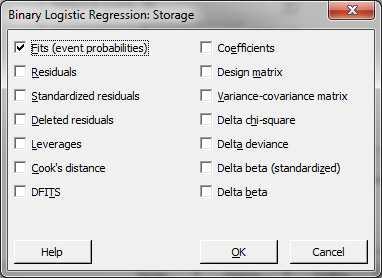3. Click “OK” in the window of “Binary Logistic Regression– Storage.”
4. Click “OK” in the window of “Binary Logistic Regression.”
5. A column of the predicted event probability is added to the data table with the heading “FITS”.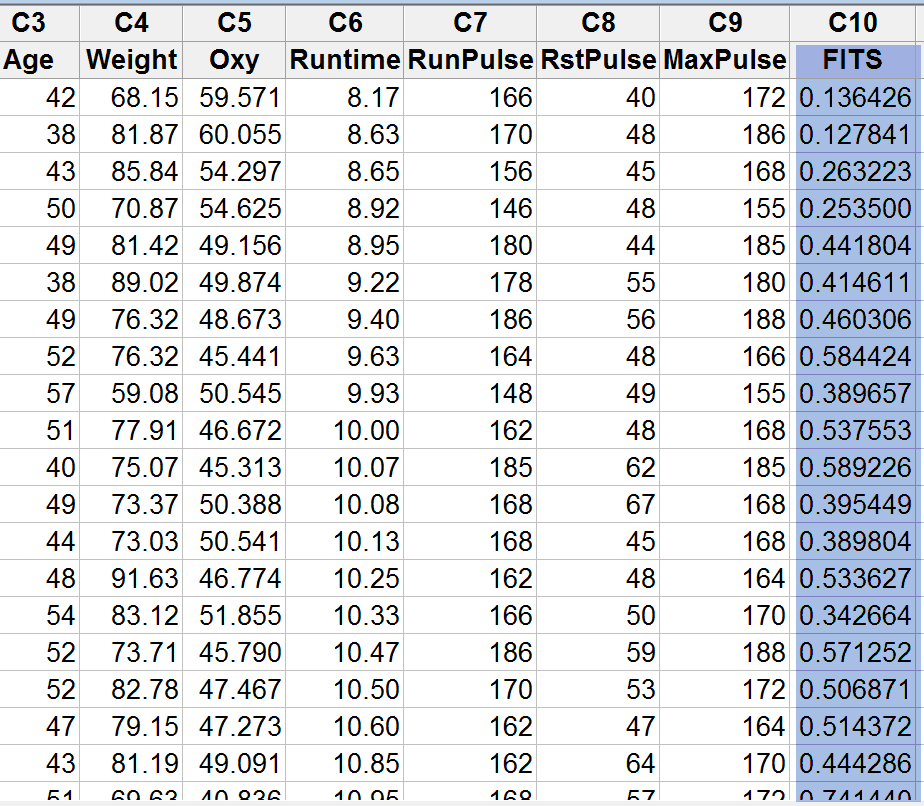Model summary: In column C10, Minitab provides the probability that the sex is male based on the only statistically significant independent variable “Oxy”.